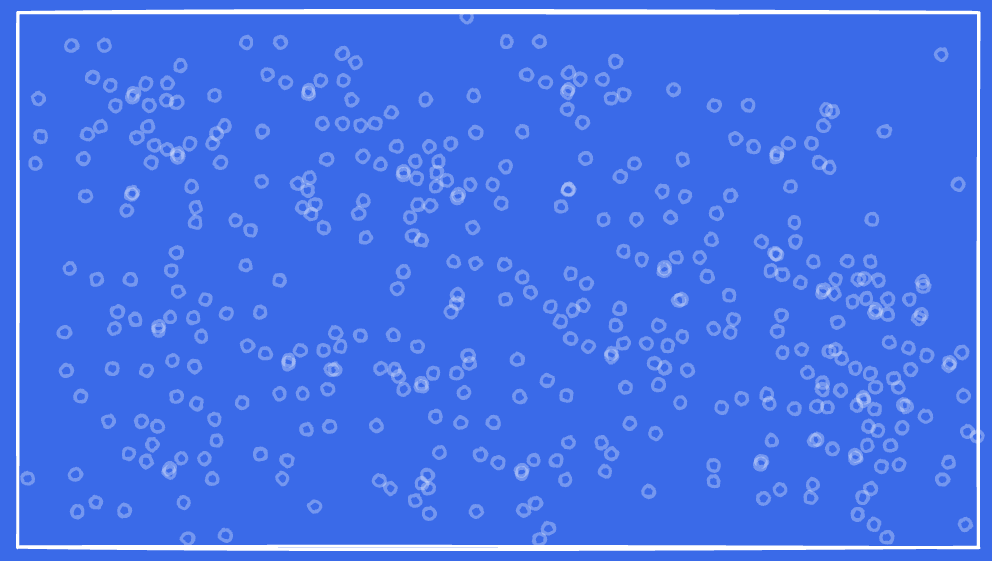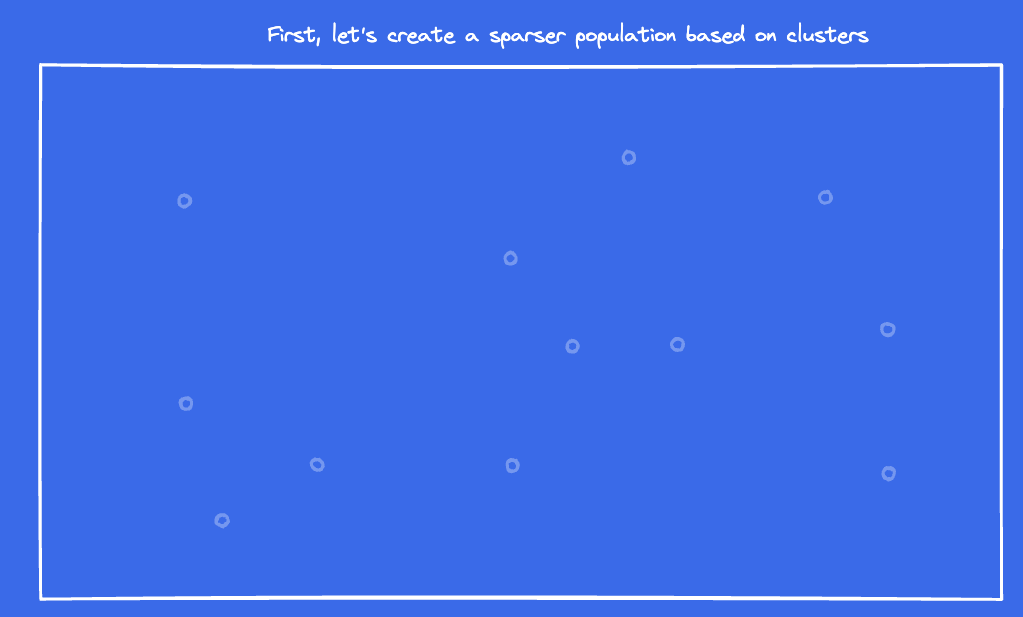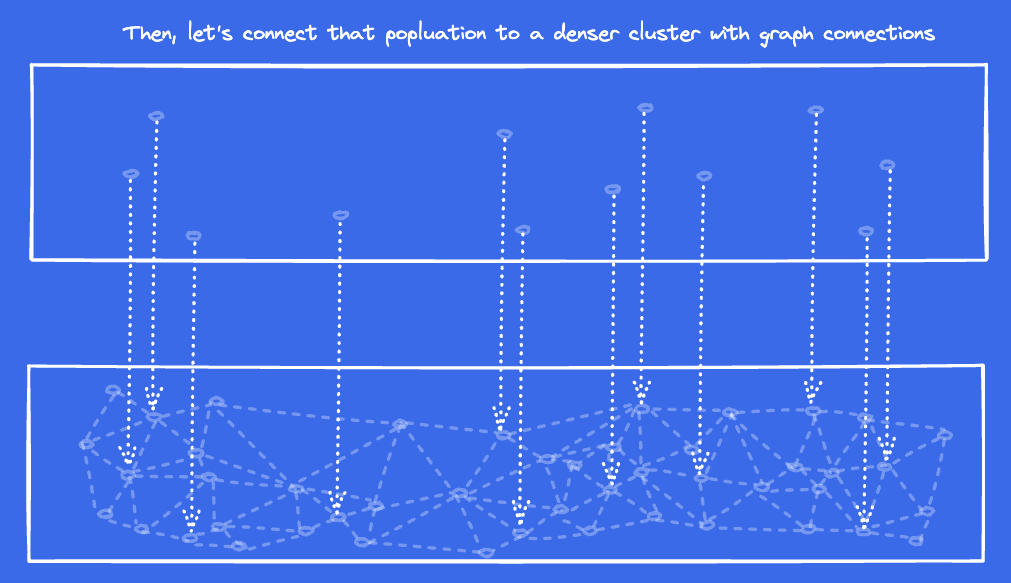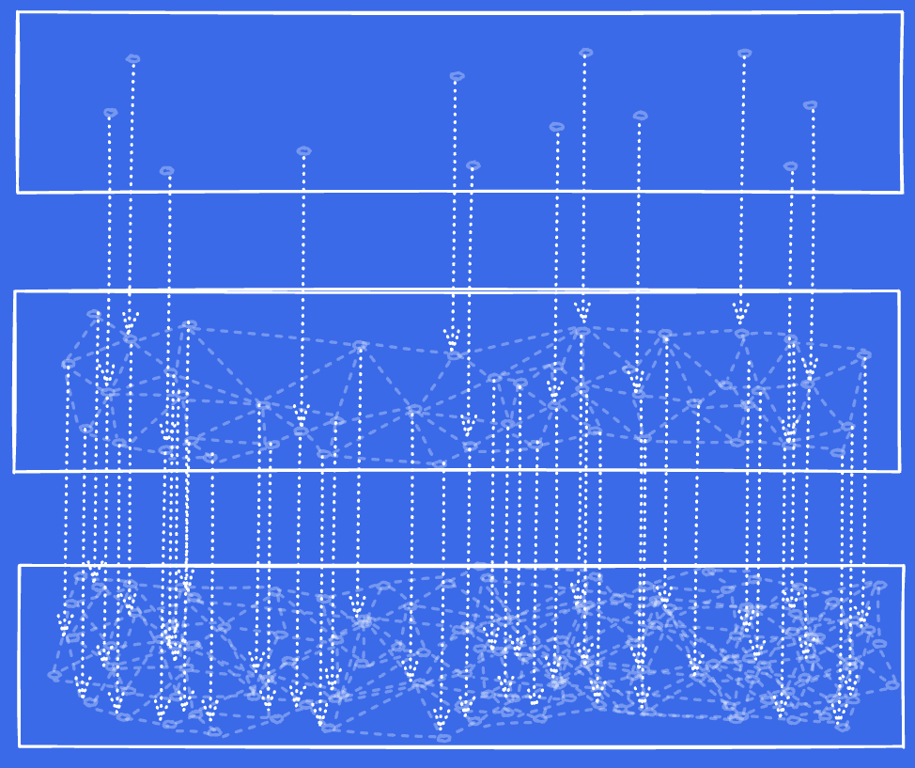# HNSW Indexes with Postgres and pgvector

Postgres’ pgvector extension recently added HNSW as a new index type for vector data. This levels up the database for vector-based embeddings output by AI models. A few months ago, we had written about approximate nearest neighbor pgvector performance using the available list-based indexes. Now, with the addition of HNSW, pgvector can use the latest graph based algorithms to approximate nearest neighbor queries. As with all things databases, there are trade-offs, so don’t throw away the list-based methodologies — and don’t throw away the techniques we discussed in scaling vector data.

## TL;DR:

HNSW is cutting edge for all vector based indexing. To build an HNSW index, run something like the following:

``````CREATE INDEX ON recipes
USING hnsw (embedding vector_l2_ops)
WITH (m = 4, ef_construction = 10);
``````

These indexes will:

• use approximations (not precision)
• be more performant than list-based indexes
• require longer index build times
• and require more memory

• Indexes will take longer to build depending on values for m and ef_construction. When increased, these values will slow the speed of index build drastically, while not improving performance. Yet, it may increase accuracy of response.
• To search more than 40 nearest neighbors, increase this `SET hnsw.ef_search = x;` value. Where `x` is the value of nearest neighbors you want to return.
• Not all queries will work with HNSW. As we said in the vector performance blog post, use `EXPLAIN` to ensure your query is using the index. If it is not using the index, simplify your query until it is, then build back to your complexity.

## What is HNSW?

HNSW is short for Hierarchical Navigable Small World. But, HNSW isn’t just one algorithm — it’s kind of like 3 algorithms in a trench coat. The first algorithm was first presented in a paper in 2011. It used graph topology to find the vertex (or element) with the local minimum nearest neighbor. Then, a couple more papers were published, but the one in 2014 combined use of multiple small worlds (i.e. hierarchy) + probability distributions + graph traversal to approximate nearest neighbors. Think of all of these algorithms as layers of graphs that get progressively more detailed.

Let’s walk through a bit of an illustration. This illustration is important to understand how tuning parameters affect performance and resource usage later. First off, imagine a 1536 dimensional universe points spread throughout a universe, and we want to find the nearest 5 points. That’s tough to think about, right? Instead, cut that down to a 2-dimensional plane. Imagine the following image as the known population of values. Place your finger somewhere on this image and then imagine trying to find the nearest 5 neighbors from this population:If you were querying nearest neighbor from a list in a database, would you iterate over all known points and measure distance? If so, that is the most costly algorithm. How can we optimize this? First, let’s create a sparse population of just 12 points:Place your finger in the same place on the image above and find the 5 closest points. Next, let’s add an additional layer that connects the points from the sparse layer to points with a denser layer constructed as a graph of points.From the top layer in the diagram above, take your 10 closest points, and trace the arrows to the 10 closest points in the bottom layer. Then, traverse all connecting lines (in graph theory, they are called edges) to connecting points (vertices). While comparing distance, only keep a list of the 10 closest -- discard any that are farther away than the top-10. (this 10 value will be associated with the ef values we discuss later).

Then, let’s add another layer, and do the same thing one more time:As you can see the illustration gets a bit messy, but the understanding is still there, I hope. The next layer repeats the same algorithm with denser data. Navigate to the points connecting to the densest layer, traverse their graphs, and run the top-10 algorithm once again. Once those are compared, extract the top-5 from the list.

You can see where the “Hierarchical Navigable Small Worlds” name comes from. It’s a hierarchy in that the series of data gets denser and denser. It’s navigable in that you can move from sparse to dense. These multiple small worlds lead to fewer calculations to get to the approximate answer. Previously, I said that it’s actually 3 algorithms in a trench coat. Arriving at the complete HNSW algorithm piggy-backed on the success of the prior.

The code for HNSW is pretty amazing. It’s clean enough that even non-C programmers can follow along to see the logic of building indexes and scanning indexes, see these links for a peek at the underlying code:

After you understand the HNSW thesis, you can go back and read the HnswSearchLayer function for fun. Additionally, see how the HNSW implementation calculates and caches distances

HNSW is much faster to query than the traditional list-based query algorithm. This performance is because the use of graphs and layers reduces the number of distance comparisons that are being run. And because fewer distance comparisons, we can run more queries concurrently as well.

The most obvious trade off for HNSW indexes is that they are approximations. But, this is no different than any existing vector index, so aside from a table-scan of comparisons. If you need absolutes, it is best to run the non-indexed query that calculates distance for each row.

The second trade-off for HNSW indexes is they can be expensive to build. The two largest contributing variables for these indexes are: size of the dataset and complexity of the index. For moderate datasets of > 1M rows, it can take 6 minutes to build some of the simplest of indexes. During that time, the database will use all the RAM it has available in `maintenance_work_mem`, while redlining the CPU. Long-story short, test it on a production-size dataset before embarking.

The third trade-off for HNSW indexes is that they are sizable — the index for 1M rows of AI embeddings can be 8GB or larger. For performance reasons, you’ll want all of this index in memory. HNSW is fast because it uses resources.

## Index tuning values

In the illustrations above, we showed how the index progressed through executing a query. But how are these indexes built? Think of index build for HNSW as a massive query that pre-calculates a larger number of distances. Index tuning is all about how the database limits the algorithms to build those indexes. Go back to the initial illustration and ask the question “how do we build an HNSW index from the data set?”

Points are saved to the top and middle layer based on probability: 1% are saved to the top layer, and 5% are saved to the middle layer. To build the index, the database loops through all values. As it loops to the next value, the algorithm uses the previously built index and the same algorithm described above to place the value within the graph. When building, each point needs to be on the graph, thus each point needs to connect to the nearest points it can find. On large datasets, it would be impossible to scan all rows and connect them in a graph to their closet neighbors within a reasonable time — thus use the following limits to constrain the build process.

m

m is the number of connections to nearest neighbors (vertices) made per point per layer within the index. When building the graph indexes, the database is seeking nearest neighbors to build out the vertices, and m is the maximum count for that layer. By limiting the number of connections, the index limits the number of connections between points at that layer. Thus, the build time improves with smaller values of m. All you have to know is that in the original paper, as m approaches infinity, it creates “graphs with polylogarithmic complexity”.

ef_construction

ef_construction is candidate list-size used during index build. Above, in the illustration, I was telling you to keep a list of 10 closet, and discard any outside those 10. During index build, the database walks through each record placing that record’s values within the index structure. Later records use the index that is currently built to more quickly position the currently processed record. As the index build process moves through the layers, it keeps a list of closest values from the prior layer. During the build, the list is sorted by distance and truncated at a length of ef_construction’s value. Once the value is placed within the graph, this list will be truncated to the length of m. The relationship between ef_construction and m is the reason ef_construction is required to be 2x the value of m. The larger the value for ef_construction the slower the index build.

What is the best values for m and ef_construction? In our tests, we have confirmed the statements from the original paper:

The only meaningful construction parameter left for the user is M. A reasonable range of M is from 5 to 48. Simulations show that smaller M generally produces better results for lower recalls and/or lower dimensional data, while bigger M is better for high recall and/or high dimensional data.

And for ef_construction:

Construction speed/index quality tradeoff is controlled via the efConstruction parameter. (…) Further increase of the efConstruction leads to little extra performance but in exchange of significantly longer construction time.

So, long-story short, keep the numbers relatively small because the quality improvement isn’t worth the performance hit.

## Query tuning values:

ef_search

This value functions the same as the ef_construction value, except for queries. This is a query-time parameter that limits the number of nearest neighbors maintained in the list. Because of this, ef_search functions as 2 limiters: maximum number of records returned and limitation on accuracy. If you are trying to return 100 nearest neighbors and the ef_search value is set to 40 (which is the default), then the query will only be capable of returning 40 rows. ef_search also limits accuracy, as nested graphs will not be traversed beyond the count. Thus, relying on few data points for comparison.

A smaller ef_search value will result in faster queries at the risk of inaccuracy. You can set it for a session as below, or use `SET LOCAL` to constrain to a transaction.

``````SET hnsw.ef_search = 5;
``````

## Using HSNW - A code sample

For this code sample, we will continue to use the SQL code found within the Postgres AI Tutorial. Pull down that file, and load it into a Postgres database with the pgvector extension. If you do not have a database with the pgvector extension, try Crunchy Bridge for your Postgres hosting and install the extension there. To load the file, run:

``````bash> cat recipe-tracker.sql | psql 'postgres://user@password:host:port/database'
``````

This schema is a set of recipes from the Armed Services Recipe list. We have categorized these recipes using OpenAI as defined in Postgres + AI blog post in this series. Then, connect to your Postgres database and run this query:

``````SELECT
name
FROM
recipes
ORDER BY
embedding <-> (
SELECT
embedding
FROM
recipes
WHERE
id = 151 ) 		-- 151 is the primary key for chocolate chip cookies
LIMIT 5;
``````

The response should be the following:

``````name
------------------------------
Bar, toffee, crisp
``````

If you prepend `EXPLAIN` to the select statement, you’ll see that the query iterated over 720 rows with on the sort statement:

``````QUERY PLAN
-----------------------------------------------------------------------------------------------
Limit  (cost=2754.87..2754.89 rows=10 width=30)
InitPlan 1 (returns \$0)
->  Index Scan using recipes_pkey on recipes recipes_1  (cost=0.28..8.31 rows=1 width=18)
Index Cond: (id = 151)
->  Sort  (cost=2746.56..2748.36 rows=720 width=30)
Sort Key: ((recipes.embedding <-> \$0))
->  Seq Scan on recipes  (cost=0.00..2731.00 rows=720 width=30)
(7 rows)
``````

Let’s create an index to see how much we can reduce the table scans:

``````CREATE INDEX
ON recipes USING hnsw (embedding vector_l2_ops) WITH (m = 4, ef_construction = 10);
``````

Now, if you run the same query again, you’ll see that that response is the same as above. With larger data sets, the rows will return sightly different rows due to the effect of approximation:

``````name
--------------------------
Bar, toffee, crisp
``````

To see that the query is using the index, you’ll see that the index scan lists using `recipes_embedding_idx` index on the next to-last row:

``````QUERY PLAN
--------------------------------------------------------------------------------------------------
Limit  (cost=100.49..118.22 rows=5 width=30)
InitPlan 1 (returns \$0)
->  Index Scan using recipes_pkey on recipes recipes_1  (cost=0.28..8.31 rows=1 width=18)
Index Cond: (id = 151)
->  Index Scan using recipes_embedding_idx on recipes  (cost=92.18..2645.18 rows=720 width=30)
Order By: (embedding <-> \$0)
``````

As listed in the TL;DR, the index optimizer is not perfect with HNSW, and prefers a simpler query. If we run a similar query but include a CTE, the HNSW index is not used by the optimizer:

``````EXPLAIN WITH random_recipe AS
(
SELECT
id,
embedding
FROM
recipes
WHERE
recipes.id = 151 LIMIT 5
)
SELECT
recipes.id,
recipes.name
FROM
recipes,
random_recipe
WHERE
recipes.id != random_recipe.id
ORDER BY
recipes.embedding <-> random_recipe.embedding LIMIT 5;
``````

Long-story short, the simpler the better for HNSW usage.

## HNSW indexes and scaling

HNSW indexes are much more performant than the older list-based indexes. They also use more resources. Concurrency is improved, but many of the processes we laid out in the Scaling PGVector blog post are still applicable.

1. Physical separation of data: because of the build requirements of the indexes, continue to host your vector data on a physically separate database.
2. Caching: if you are running the same queries many times with very little change to the data, consider using caching.
3. Dimensional Reduction: dimensional reduction is even better with HNSW indexes. If your dataset is one that works well with dimensional reduction, you can benefit from faster build times and small indexes and improved query times.Written by

Christopher Winslett

September 1, 2023 More by this author